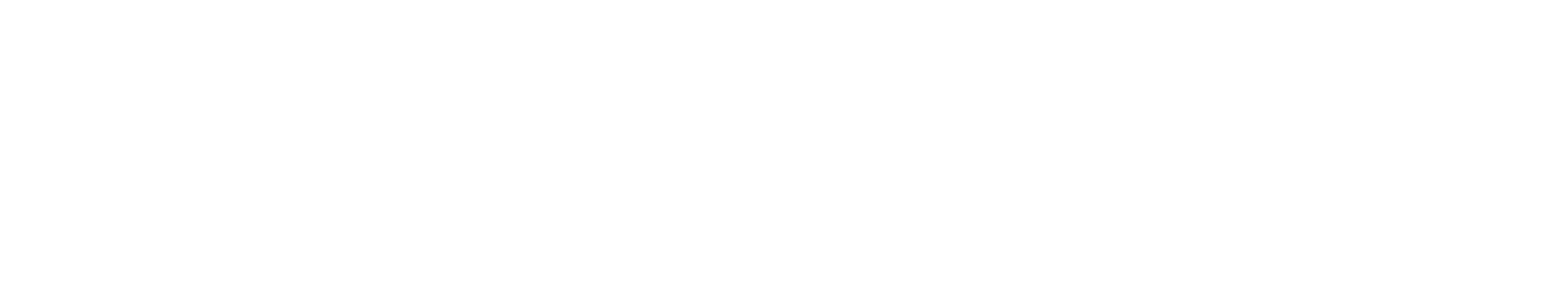﻿GeoFence.Drawer.Draw Method# GeoFenceAddLanguageSpecificTextSet("LSTDC374747_0?cpp=::|nu=.");DrawerAddLanguageSpecificTextSet("LSTDC374747_1?cpp=::|nu=.");Draw Method

Draws line from the initial or last drawn point.

Namespace:  CoordinateSharp
Assembly:  CoordinateSharp (in CoordinateSharp.dll) Version: 2.10.2.2Syntax
C#
```public void Draw(
Distance d,
double bearingChange
)```

#### Parameters

d
Type: CoordinateSharpDistance
Distance
bearingChange
Type: SystemDouble
Bearing change in degreesExamples
The following example draws a 5 km square in the USA.
```//Create a coordinate with EagerLoading off for efficiency during drawing.
Coordinate c = new Coordinate(31.65, -84.02, new EagerLoad(false));

//Create the GeoFence Drawer, specifying an ellipsoidal earth
//shape with a start bearing / direction faced of 0 degrees.
GeoFence.Drawer gd = new GeoFence.Drawer(c, Shape.Ellipsoid, 0);

//Draw the first line using the initial bearing (0 means no change in heading)
gd.Draw(new Distance(5), 0);
//Draw the next to line by changing 90 degrees at each point.
gd.Draw(new Distance(5), 90);
gd.Draw(new Distance(5), 90);
//Close the shape by drawing from the line end point to the initial coordinate.
gd.Close();```See Also